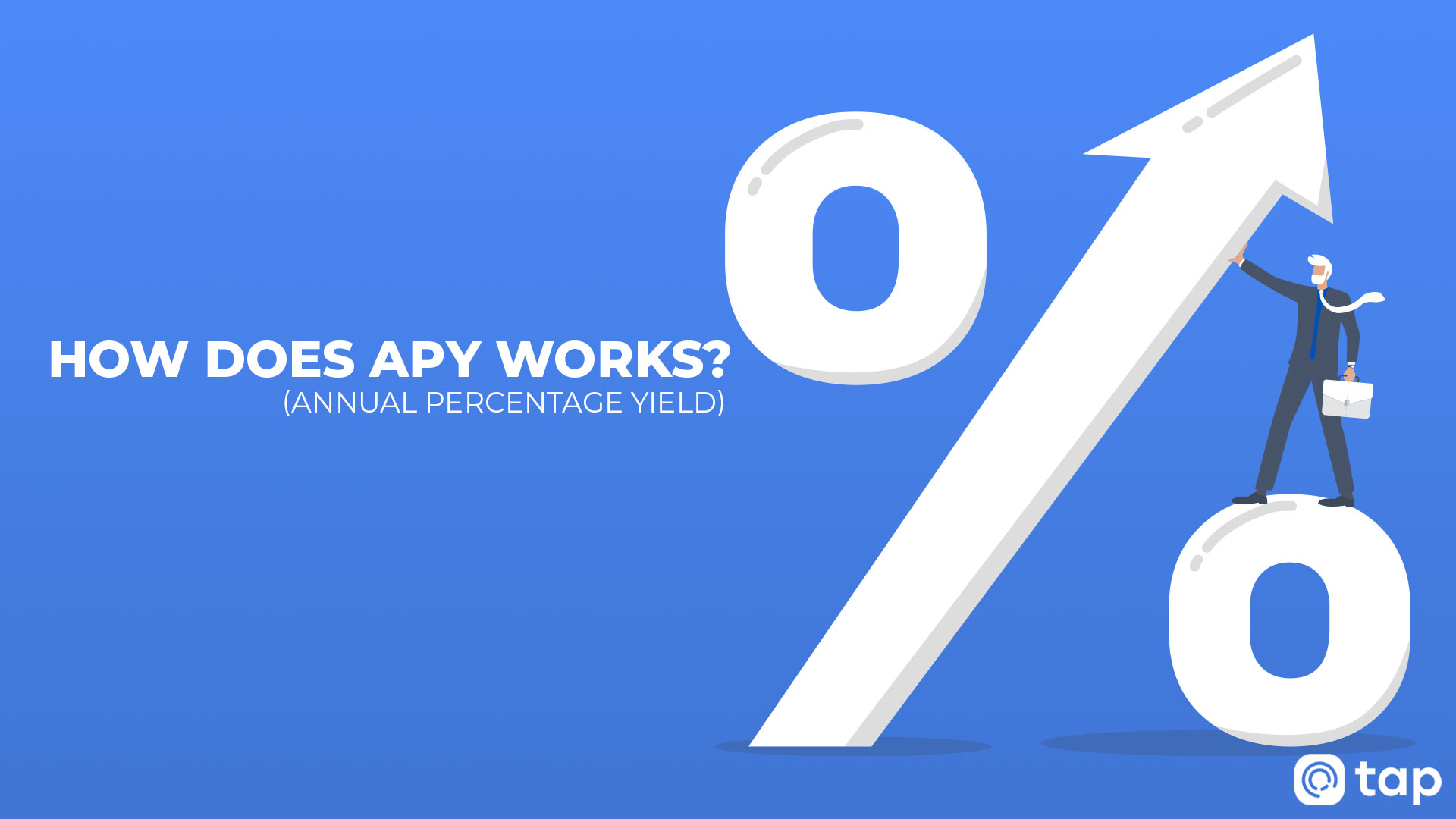# How Does APY ( Annual Percentage Yield ) Work?

So you decided to go deeper into the fundamentals of investing and learn what an APY is. You've come to the right place, let's get you started with this perplexing "APY" term.

What Is APY?

In conventional finance, a savings account frequently offers both a low-interest rate and an annual percentage yield (APY). Let's look at what they are and what they mean.

• The Annual Percentage Yield (APY) is the annual return from the principal and accumulated interest on investments or savings, expressed as a percentage.
• The simple interest rate is the amount earned on the original deposit.

Assume an account at a bank offers a yearly interest rate of 5%. If someone deposits €2,000 into the account, it will be worth €2,100 after a year with the 5% yearly interest rate.

The Difference Between Interest Rate, APY and APR

The APY takes into account the impact of compounding, whereas the interest rate does not. The APY is the projected rate of return earned annually on a deposit after taking compound interest into account.

Compounding interest is the interest that a person accrues from their initial deposit, as well as the interest they earn from their original investment (or in other words, the initial deposit amount plus the interest generated).

The terms APY and APR are frequently used interchangeably, although they represent two different things. These words are sometimes confused due to their close resemblance. However, APY and APR aren't the same things.

The APR (annual percentage rate) is a formula that determines how much interest you'll pay when borrowing money and is the rate of return earned if your funds are invested in an interest-bearing account.

When a person takes out a loan, their lender sets an APR that varies based on the loan. APRs are either fixed or fluctuating depending on the type of loan the user requires. However, the APR is a rather basic interest rate and does not take compounding into account, unlike APY.

How Is APY Calculated?

APY represents your rate of return, also known as the amount of earnings or profit you can make. Of course, your ultimate earnings will vary depending on how long you keep your assets invested while the holding period will influence how much you will earn.

APY measures the rate of the annual return earned on any amount of money or investment after taking into account compounding interest.

The following is the formula for calculating APY:

APY = (1 + p/n)ⁿ − 1

Where:

p = periodic rate of return (or annual APR)

n = number of compounding periods each year

Bear in mind that an APY can be calculated in a variety of ways depending on the provider. Tap provides flexible staking that enables users to invest or withdraw their assets at any time to earn weekly passive income.

The APY is represented in a straightforward interest format, with the weekly yield representing the interest rate that will be paid into your wallet depending on the value of your invested assets.